# Angle in a semicircle is 90 degrees

Martin McBride, 2020-08-21
Tags circle semicircle angle right angle
Categories maths gcse geometry

We can draw a triangle where the base is a diameter of a circle, and the third point of the triangle lies on the circumference of the circle: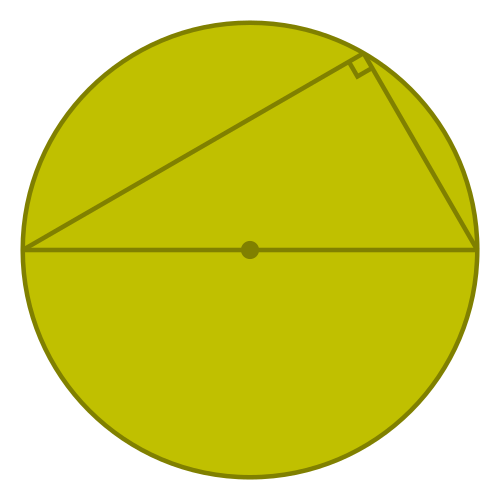The angle at the circumference (called the angles in a semicircle) is 90°.

This theorem is covered in this video on circle theorems:

## Proof

We can prove this as follows.

We label the vertices of the triangle A, B and C, and label the centre of the circle O. We are trying to prove that the angle at B is 90°.

We first draw an extra line from O to B like this: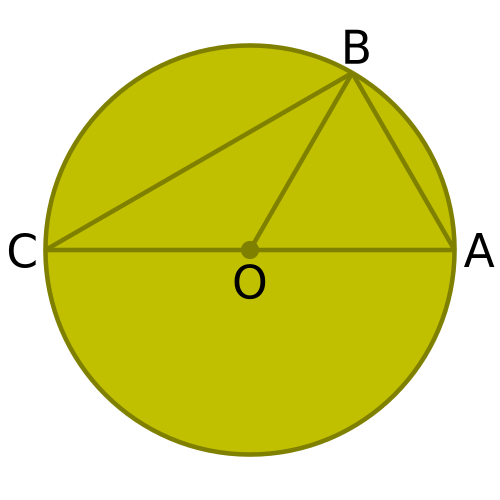Notice that the lines OA, OB and OC are all radii of the circle, and therefore all of equal length.

Looking at the triangle AOB, this is an isosceles triangle (from the rule 2 radii form an isosceles triangle). So the two angles at the circumference are equal (we will call them a):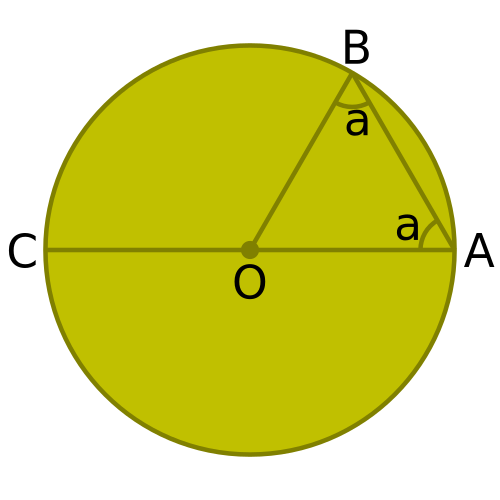By the same logic, the two angles at the circumference in triangle COB are equal, and we will call them b: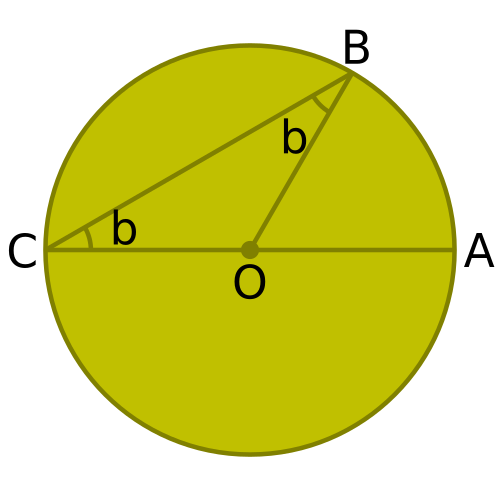Looking at the original triangle ABC: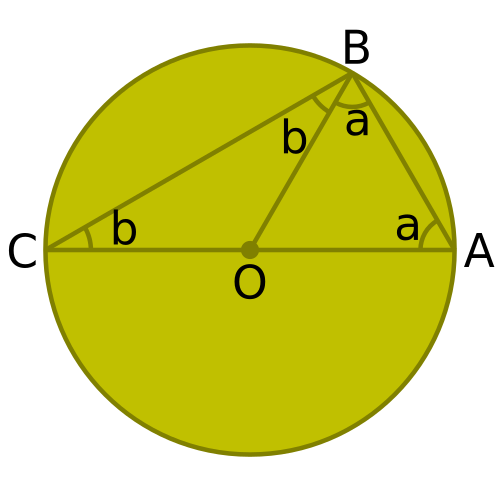The angle at A is a, the angle at B is a + b, and the angle at C is b. Since the three angles of a triangle add up to 180° we have:

a + (a + b) + b = 180°


So:

2(a + b) = 180°


Which means:

a + b = 90°


Since the angle at B is a + b, this proves that the angle at B is 90°.